CHE20028 PHYSICAL INORGANIC CHEMISTRY STATISTICAL THERMODYNAMICS LECTURE 2

• Slides: 25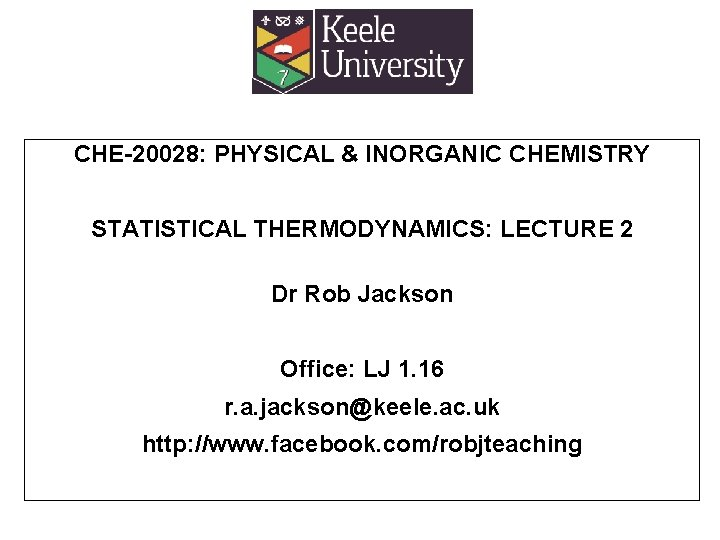CHE-20028: PHYSICAL & INORGANIC CHEMISTRY STATISTICAL THERMODYNAMICS: LECTURE 2 Dr Rob Jackson Office: LJ 1. 16 r. a. [email protected] ac. uk http: //www. facebook. com/robjteaching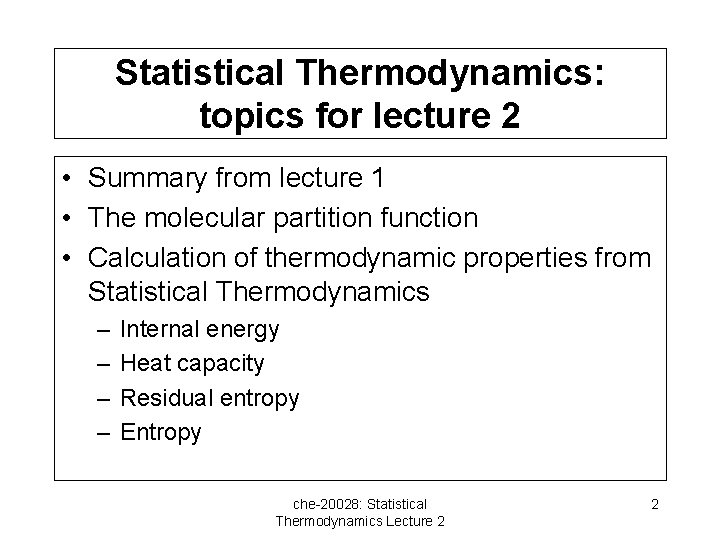Statistical Thermodynamics: topics for lecture 2 • Summary from lecture 1 • The molecular partition function • Calculation of thermodynamic properties from Statistical Thermodynamics – – Internal energy Heat capacity Residual entropy Entropy che-20028: Statistical Thermodynamics Lecture 2 2The molecular partition function- 1 • What contributes to the energy of a molecule? – Vibrational (V), translational (T) and rotational (R) modes of motion – Electron distribution (E) – Electronic and nuclear spin (S) che-20028: Statistical Thermodynamics Lecture 2 3The molecular partition function- 2 • Remembering from lecture 1 that the partition function q is given by: • We can substitute for Ei in this expression: che-20028: Statistical Thermodynamics Lecture 2 4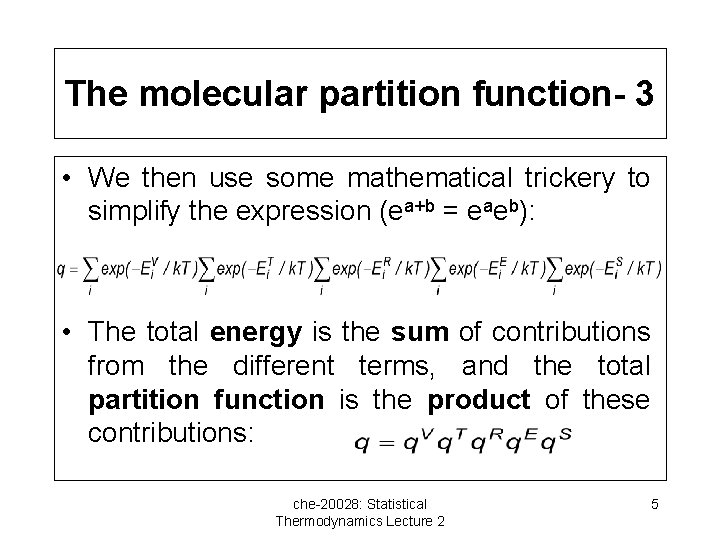The molecular partition function- 3 • We then use some mathematical trickery to simplify the expression (ea+b = eaeb): • The total energy is the sum of contributions from the different terms, and the total partition function is the product of these contributions: che-20028: Statistical Thermodynamics Lecture 2 5Note on electron and spin partition functions • While it is not possible to obtain expressions for these, as we have done for the other terms, we note the following: – For closed shell molecules, excited states are so high in energy that only the ground state is occupied, and q. E= 1 – Electron spin makes an important contribution when there are unpaired electrons, since the electron can occupy either spin state, and then q. S=2 che-20028: Statistical Thermodynamics Lecture 2 6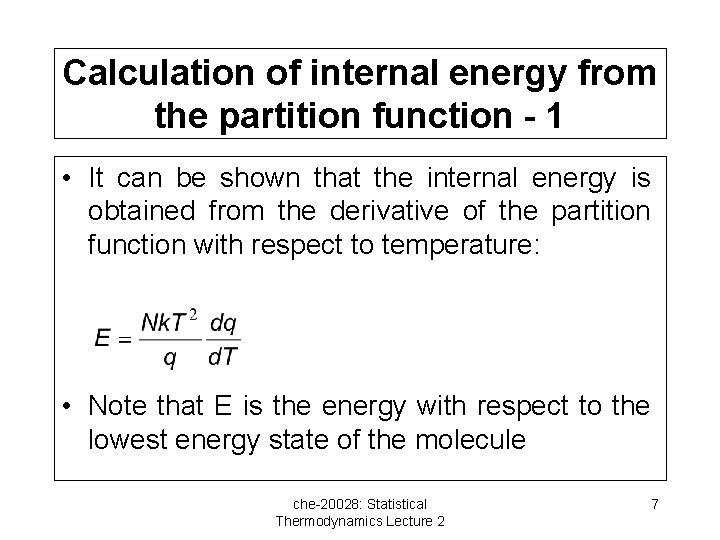Calculation of internal energy from the partition function - 1 • It can be shown that the internal energy is obtained from the derivative of the partition function with respect to temperature: • Note that E is the energy with respect to the lowest energy state of the molecule che-20028: Statistical Thermodynamics Lecture 2 7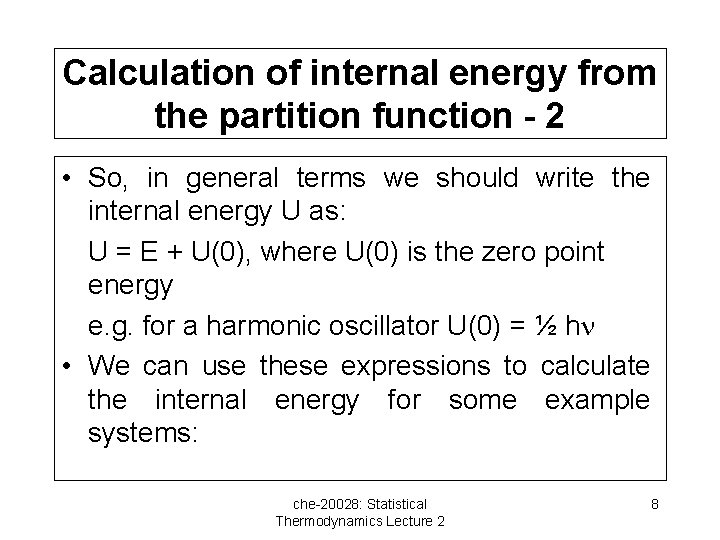Calculation of internal energy from the partition function - 2 • So, in general terms we should write the internal energy U as: U = E + U(0), where U(0) is the zero point energy e. g. for a harmonic oscillator U(0) = ½ h • We can use these expressions to calculate the internal energy for some example systems: che-20028: Statistical Thermodynamics Lecture 2 8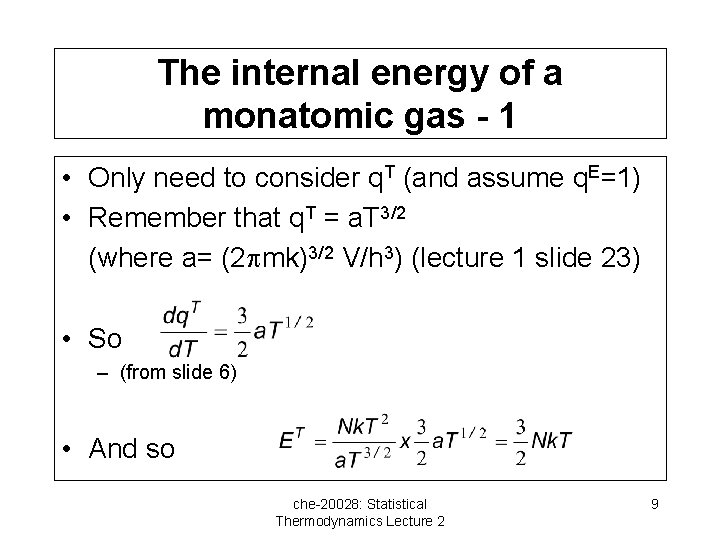The internal energy of a monatomic gas - 1 • Only need to consider q. T (and assume q. E=1) • Remember that q. T = a. T 3/2 (where a= (2 mk)3/2 V/h 3) (lecture 1 slide 23) • So – (from slide 6) • And so che-20028: Statistical Thermodynamics Lecture 2 9The internal energy of a monatomic gas - 2 • So if N=NA, ET = (3/2)RT Ø U = U(0) + (3/2)RT • Example: calculate the internal energy of Argon gas at 300 K Ø U = U(0) + (3/2) x 8. 314 x 300 J mol-1 Ø = 3741. 3 J mol-1 • What would be the result for another monatomic gas? che-20028: Statistical Thermodynamics Lecture 2 10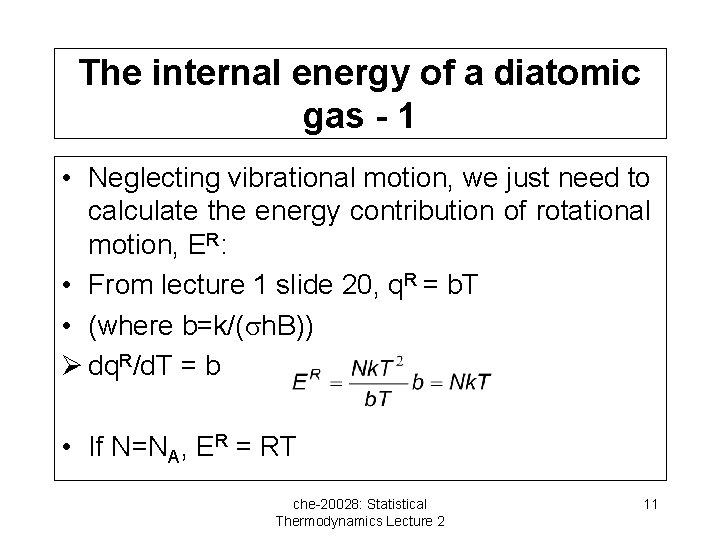The internal energy of a diatomic gas - 1 • Neglecting vibrational motion, we just need to calculate the energy contribution of rotational motion, ER: • From lecture 1 slide 20, q. R = b. T • (where b=k/( h. B)) Ø dq. R/d. T = b • If N=NA, ER = RT che-20028: Statistical Thermodynamics Lecture 2 11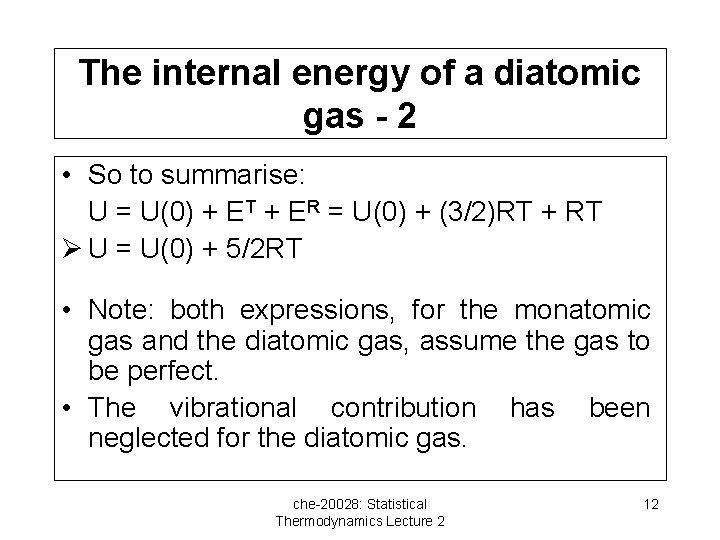The internal energy of a diatomic gas - 2 • So to summarise: U = U(0) + ET + ER = U(0) + (3/2)RT + RT Ø U = U(0) + 5/2 RT • Note: both expressions, for the monatomic gas and the diatomic gas, assume the gas to be perfect. • The vibrational contribution has been neglected for the diatomic gas. che-20028: Statistical Thermodynamics Lecture 2 12How can we make comparisons with experimental values? • Heat capacities can be calculated, and compared with experimental values. • Remember that CV= d. U/d. T at constant volume. – For a monatomic gas: U = U(0) + (3/2)RT, d. U/d. T= (3/2)R= 12. 47 J mol-1 K-1 – For a diatomic gas: U = U(0) + (5/2)RT, d. U/d. T= (5/2)R= 20. 79 J mol-1 K-1 che-20028: Statistical Thermodynamics Lecture 2 13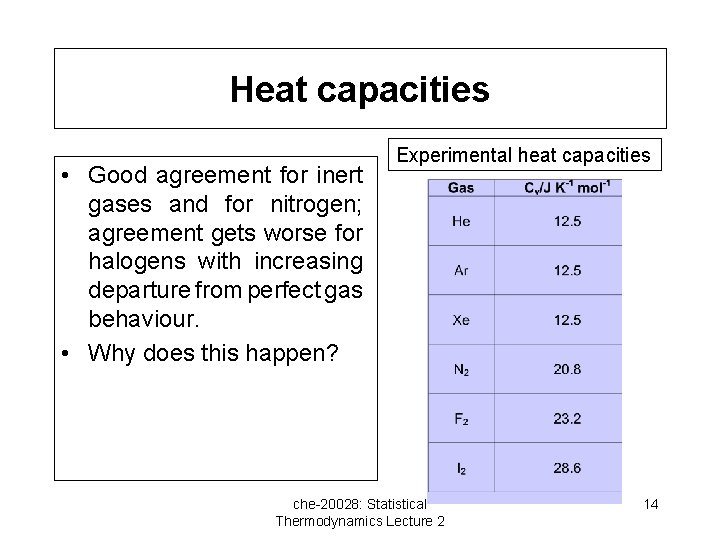Heat capacities • Good agreement for inert gases and for nitrogen; agreement gets worse for halogens with increasing departure from perfect gas behaviour. • Why does this happen? Experimental heat capacities che-20028: Statistical Thermodynamics Lecture 2 14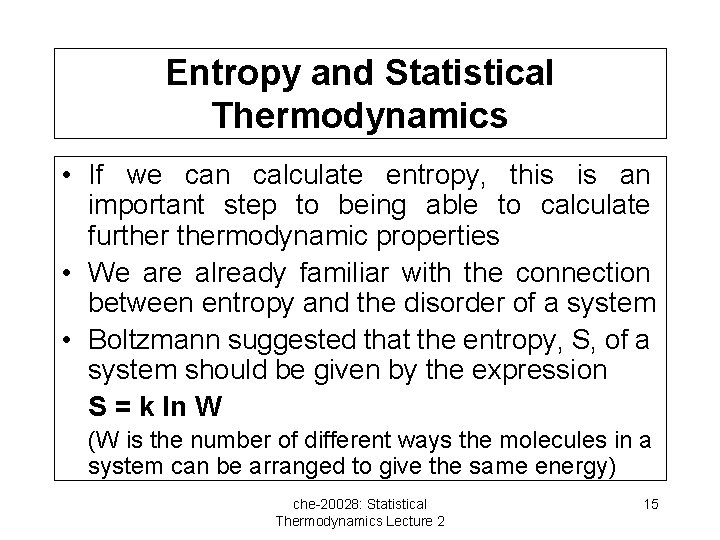Entropy and Statistical Thermodynamics • If we can calculate entropy, this is an important step to being able to calculate furthermodynamic properties • We are already familiar with the connection between entropy and the disorder of a system • Boltzmann suggested that the entropy, S, of a system should be given by the expression S = k ln W (W is the number of different ways the molecules in a system can be arranged to give the same energy) che-20028: Statistical Thermodynamics Lecture 2 15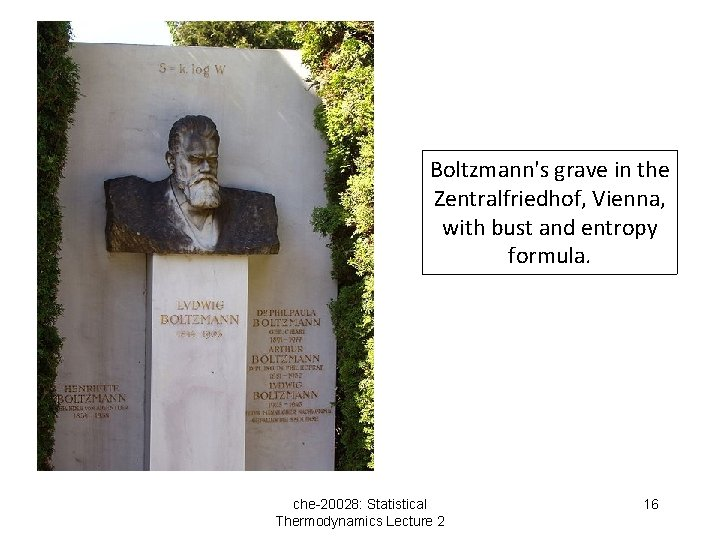Boltzmann's grave in the Zentralfriedhof, Vienna, with bust and entropy formula. che-20028: Statistical Thermodynamics Lecture 2 16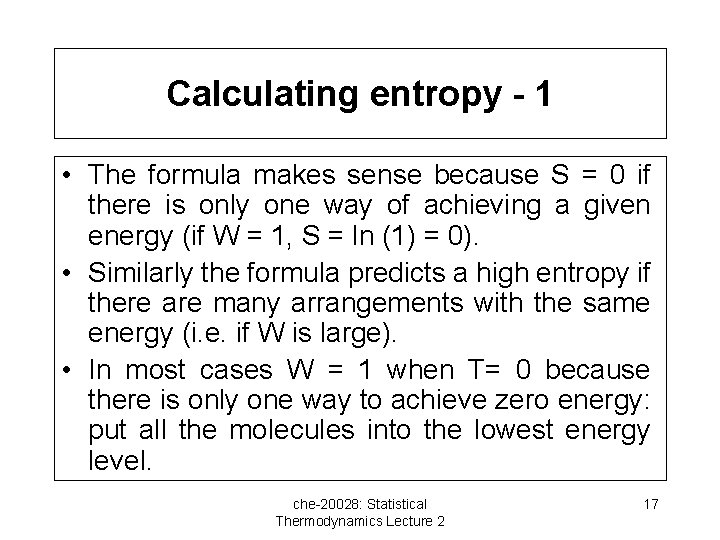Calculating entropy - 1 • The formula makes sense because S = 0 if there is only one way of achieving a given energy (if W = 1, S = ln (1) = 0). • Similarly the formula predicts a high entropy if there are many arrangements with the same energy (i. e. if W is large). • In most cases W = 1 when T= 0 because there is only one way to achieve zero energy: put all the molecules into the lowest energy level. che-20028: Statistical Thermodynamics Lecture 2 17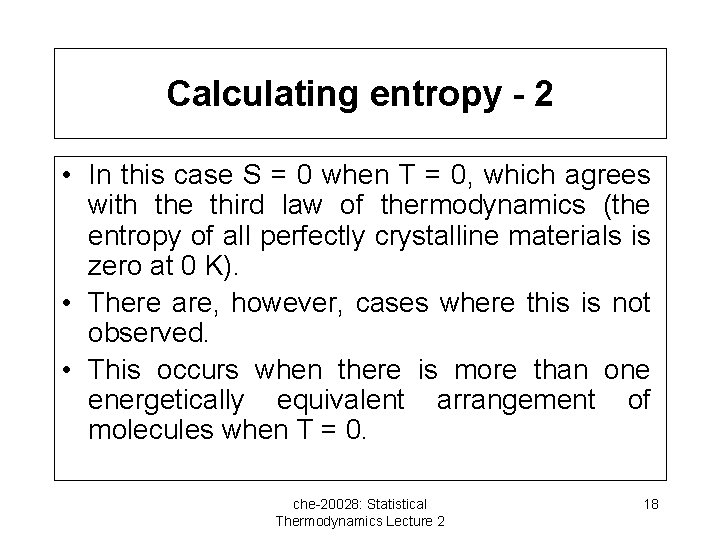Calculating entropy - 2 • In this case S = 0 when T = 0, which agrees with the third law of thermodynamics (the entropy of all perfectly crystalline materials is zero at 0 K). • There are, however, cases where this is not observed. • This occurs when there is more than one energetically equivalent arrangement of molecules when T = 0. che-20028: Statistical Thermodynamics Lecture 2 18Residual entropy - 1 • There are several examples of molecules that can have more than one energetically equivalent arrangement at 0 K – Examples include linear molecules where they may be no energetic difference between the arrangement AB AB and the arrangement AB BA AB AB – e. g. solid carbon monoxide, where there is no energetic difference between the arrangements CO CO and CO CO OC CO che-20028: Statistical Thermodynamics Lecture 2 19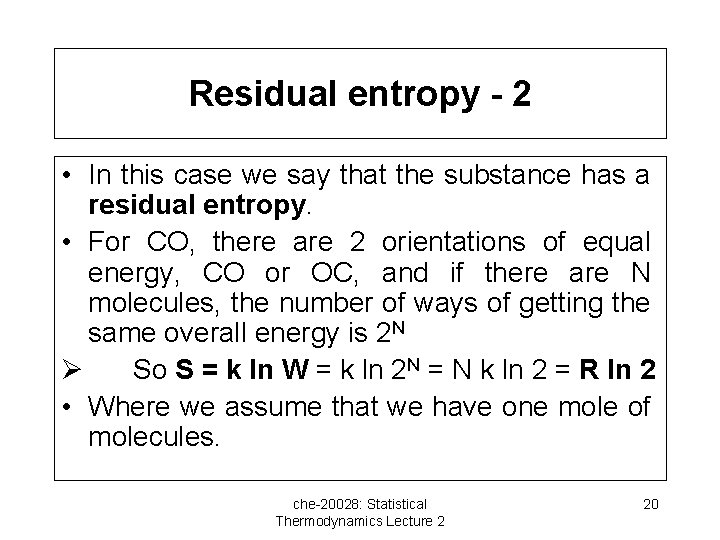Residual entropy - 2 • In this case we say that the substance has a residual entropy. • For CO, there are 2 orientations of equal energy, CO or OC, and if there are N molecules, the number of ways of getting the same overall energy is 2 N Ø So S = k ln W = k ln 2 N = N k ln 2 = R ln 2 • Where we assume that we have one mole of molecules. che-20028: Statistical Thermodynamics Lecture 2 20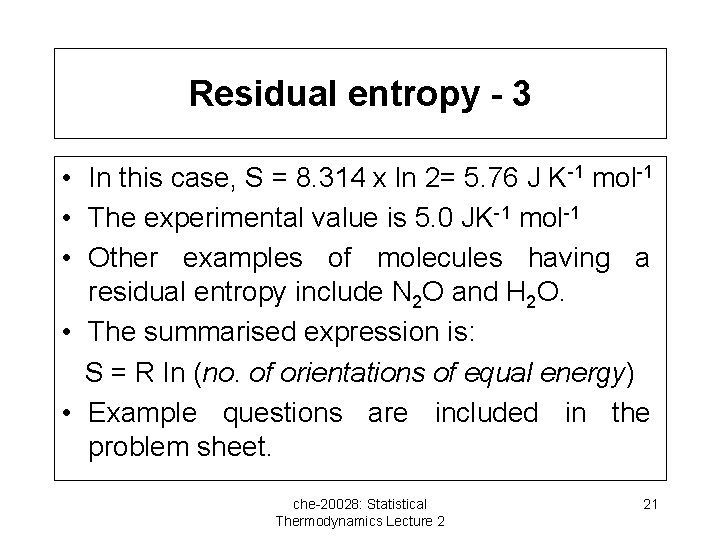Residual entropy - 3 • In this case, S = 8. 314 x ln 2= 5. 76 J K-1 mol-1 • The experimental value is 5. 0 JK-1 mol-1 • Other examples of molecules having a residual entropy include N 2 O and H 2 O. • The summarised expression is: S = R ln (no. of orientations of equal energy) • Example questions are included in the problem sheet. che-20028: Statistical Thermodynamics Lecture 2 21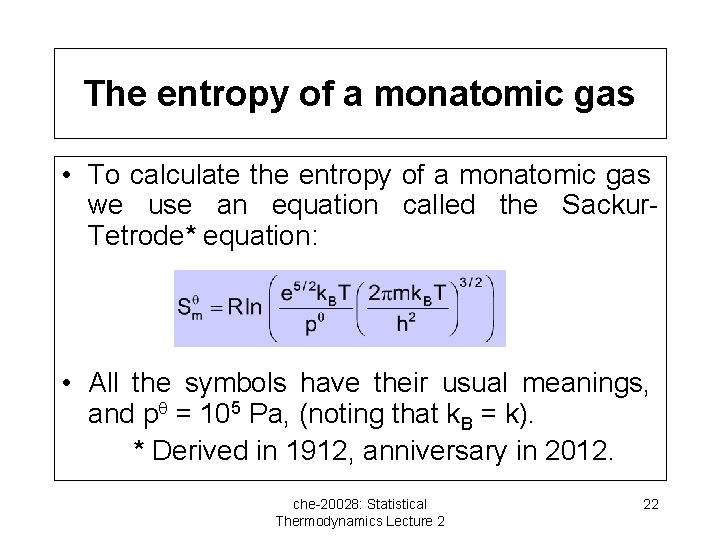The entropy of a monatomic gas • To calculate the entropy of a monatomic gas we use an equation called the Sackur. Tetrode* equation: • All the symbols have their usual meanings, and p = 105 Pa, (noting that k. B = k). * Derived in 1912, anniversary in 2012. che-20028: Statistical Thermodynamics Lecture 2 22Sackur-Tetrode equation example • Calculate the molar entropy of argon gas (M = 39. 948 g mol-1) at 298 K and 105 Pa Divide the calculation into sections and rewrite the equation as: Sm = R ln (A B 3/2) A = e 5/2 k. T/p = 12. 182 x 1. 381 x 10 -23 x 293 /105 = 5. 014 x 10 -25 B = 2 mk. T/h 2 = 2 x (39. 948 x 1. 661 x 10 -27) x 1. 381 x 10 -23 x 298)/(6. 626 x 10 -34)2 = 3. 908 x 1021, so B 3/2 = 2. 443 x 1032 S = R ln (5. 014 x 10 -25 x 2. 443 x 1032) = 154. 84 J mol-1 K-1 (Experimental value is 154. 6 J mol-1 K-1) che-20028: Statistical Thermodynamics Lecture 2 23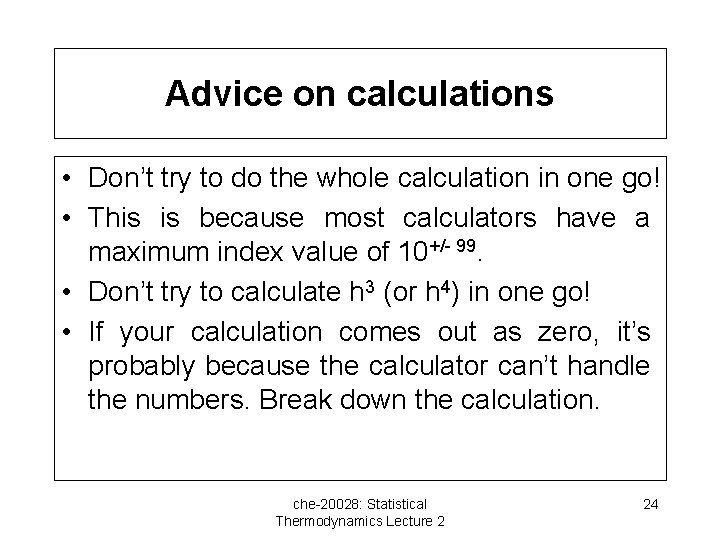Advice on calculations • Don’t try to do the whole calculation in one go! • This is because most calculators have a maximum index value of 10+/- 99. • Don’t try to calculate h 3 (or h 4) in one go! • If your calculation comes out as zero, it’s probably because the calculator can’t handle the numbers. Break down the calculation. che-20028: Statistical Thermodynamics Lecture 2 24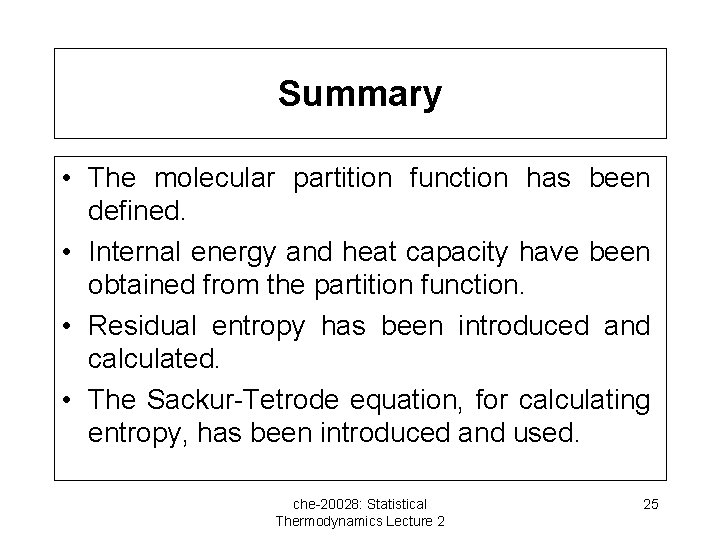Summary • The molecular partition function has been defined. • Internal energy and heat capacity have been obtained from the partition function. • Residual entropy has been introduced and calculated. • The Sackur-Tetrode equation, for calculating entropy, has been introduced and used. che-20028: Statistical Thermodynamics Lecture 2 25